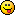Discussion about math, puzzles, games and fun.   Useful symbols: ÷ × ½ √ ∞ ≠ ≤ ≥ ≈ ⇒ ± ∈ Δ θ ∴ ∑ ∫  π  -¹ ² ³ °

You are not logged in.

## #1 Re: Help Me ! » Help needed with a simple question. » 2013-08-10 09:30:05

Thank you both very much for your patience . I can see I have a long road ahead of me to understand all of that. At least I know what to look for now.

## #2 Re: Help Me ! » Help needed with a simple question. » 2013-08-10 08:53:33

As you can see from the above drawing, each triangle is extrapolated from the one below.
All measurements in cm.
if you were given the first measurement, eg 15 cm, how would you express a formula to calculate the rest.
Yes I know if I did not round it all to 2 decimal place it would not work. The point is I don't need .o1 of a cm accuracy to build this in the real world. Can this sort of 'error' be built into a math formula?

## #3 Re: Help Me ! » Help needed with a simple question. » 2013-08-10 08:46:59

This is the drawing for half of a window frame to be welded up.

## #4 Re: Help Me ! » Help needed with a simple question. » 2013-08-10 07:57:55

Would it help if I put up a quick drawing to explain what prompted the question?
I have to admit I'm not sure the real world application would stand up to a detailed formula.
The idea was to be able to work out the total design with nothing more than one measurement and a formula.However I was more interested in how such a formula would be expressed rather than if it would work. I could only get it to work by rounding everything to 2 decimal places.I'm not even sure if it would work at  larger scale.

## #5 Re: Help Me ! » Help needed with a simple question. » 2013-08-10 05:27:25

ok, if we start with an equal triangle number 1 with short sides 2m. Then the long side is 2.8m. That then becomes one of triangle number 2's short sides.so triangle number 2s long side is 4m. that then becomes a side for triangle number 3, etc etc.that creates a spiral made of triangles that increase in size in relation to the previous triangle. (I've rounded the math up a bit).
using what you put above, the closest I can get right now is (using a,b,and c )
c[n]=c[n-a+b]
but that only looks to be part of it.

## #6 Re: Help Me ! » Help needed with a simple question. » 2013-08-10 04:51:52

bobbym wrote:

If A + B = C

what about D, is it B + C?

A quick look around suggests I need to be looking into recursive,would that be right? thanks for your help, much appreciated## #7 Re: Help Me ! » Help needed with a simple question. » 2013-08-10 04:41:57

To clarify, I know the statement
" If the next A or B is the previous C, then the next C is the previous A+B"
is probably not very accurate. I guess I'm trying to find out how this would be wrote as a formula that describes how the value of A,B and C change. I know its probably childishly simple to most of you. I would be eternally grateful if someone would point me in the right direction and I'll try and learn more.

## #8 Re: Help Me ! » Help needed with a simple question. » 2013-08-10 04:30:16

yes, sorry, my mistake.

## #9 Help Me ! » Help needed with a simple question. » 2013-08-10 04:23:49

Replies: 21

Hi everyone. As i said in my intro I am a welder. I've been asked to construct a spiral made up of triangles.  Like most jobs I have sat down to draw it out and make cutting lists etc so I can give the customer a fair estimate of cost.
After doing the old A2+B2=C2 a few times to work out the lengths of the sides I notices I no longer need to do that. The answer for the next triangle is always in the previous one.
E.G If the next A or B is the previous c, then the next c is the previous a+b.(to my level of accuracy anyway lol)
This got me thinking,how would I describe this change of value in math 'talk'.
I'm more interested in the way it would be described than the lvl of accuracy.

I know this is probably a simple question and apologies if I offend anyone.
Thank you all in advance for your patience.